# Regressing thermodynamic data

### EOSregress in CHNOSZ

#### 2019-08-02

This vignette demonstrates `EOSregress()` and related functions for the regression of heat capacity and volumetric data to obtain “equations of state” coefficients.

# A note on the equations

The CHNOSZ thermodynamic database uses the revised Helgeson-Kirkham-Flowers equations of state (EOS) for aqueous species. Different terms in these equations give the “non-solvation” and “solvation” contributions to the standard molal heat capacity (CP°) and volume (V°) as a function of temperature (T) and pressure (P).

The equations were originally described by Helgeson et al. (1981Helgeson HC, Kirkham DH, Flowers GC. 1981. Theoretical prediction of the thermodynamic behavior of aqueous electrolytes at high pressures and temperatures: IV. Calculation of activity coefficients, osmotic coefficients, and apparent molal and standard and relative partial molal properties to 600°C and 5 Kb. American Journal of Science 281(10): 1249–1516. doi: 10.2475/ajs.281.10.1249); for CP° the equation is

CP° = c1 + c2 / (T - θ)2 + ωTX

Here, c1 and c2 are the non-solvation parameters, and ω is the solvation parameter. θ is equal to 228 K, and X is one of the “Born functions” that relates the solvation process to the dielectric properties of water. For neutral species, all of the parameters, including the “effective” value of ω, are constant. However, for charged species, ω has non-zero derivatives at T > 100 °C, increasing with temperature, that depend on the charge and ionic radius. Revisions by Tanger and Helgeson (1988Tanger JC IV, Helgeson HC. 1988. Calculation of the thermodynamic and transport properties of aqueous species at high pressures and temperatures: Revised equations of state for the standard partial molal properties of ions and electrolytes. American Journal of Science 288(1): 19–98. doi: 10.2475/ajs.288.1.19) and Shock et al. (1992Shock EL, Oelkers EH, Johnson JW, Sverjensky DA, Helgeson HC. 1992. Calculation of the thermodynamic properties of aqueous species at high pressures and temperatures: Effective electrostatic radii, dissociation constants, and standard partial molal properties to 1000 °C and 5 kbar. Journal of the Chemical Society, Faraday Transactions 88(6): 803–826. doi: 10.1039/FT9928800803) refined the equations to improve their high-T and P behavior.

# A note on the algorithms

The regression functions are essentially a wrapper around the `water()`-related functions in CHNOSZ and `lm()` in R. The former provide values of the Born functions. Accordingly, numerical values for all of the variables in the equations (e.g. 1/(T - θ)2 and TX) can be calculated at each experimental T and P.

Applying a linear model (`lm`), the coefficients in the model can be obtained. In this case, they correspond to the HKF parameters, e.g. `c1` (the intercept), `c2` and ω. The coefficients in this model are constants, restricing application of the model to neutral (uncharged) species, for which the high-temperature derivatives of ω are 0. Further below, a procedure is described to iteratively solve the equations for charged species.

# An example for neutral species

This is from the first example from `EOSregress()`. The `?` indicates a documentation topic in R. To view it, type `?EOSregress` or `help(EOSregress)` at the R prompt. Here, we regress experimental heat capacities of aqueous methane (CH4) using the revised HKF equations. First, load CHNOSZ and its database:

``````library(CHNOSZ)
reset()``````
```## reset: resetting "thermo" object
```
```## obigt: loading default database with 1849 aqueous, 3372 total species
```

Now, read a data file with experimental measurements from Hnědkovský and Wood (1997Hnědkovský L, Wood RH. 1997. Apparent molar heat capacities of aqueous solutions of CH4, CO2, H2S, and NH3 at temperatures from 304 K to 704 K at a pressure of 28 MPa. Journal of Chemical Thermodynamics 29(7): 731–747. doi: 10.1006/jcht.1997.0192). Here we also convert J to cal and MPa to bar:

``````file <- system.file("extdata/cpetc/Cp.CH4.HW97.csv", package = "CHNOSZ")
Cpdat\$Cp <- convert(Cpdat\$Cp, "cal")
Cpdat\$P <- convert(Cpdat\$P, "bar")``````

Next, we specify the terms in the HKF equations and perform the regression using `EOSregress()`. In the second call to `EOSregress()` below, only for T < 600 K are included in the regression. The coefficients here correspond to c1, c2 and ω in the HKF equations.

``````var <- c("invTTheta2", "TXBorn")
Cplm_high <- EOSregress(Cpdat, var)
Cplm_low <- EOSregress(Cpdat, var, T.max = 600)
Cplm_low\$coefficients``````
``````## (Intercept)  invTTheta2      TXBorn
##     38.2655 105970.9439 -29080.5286``````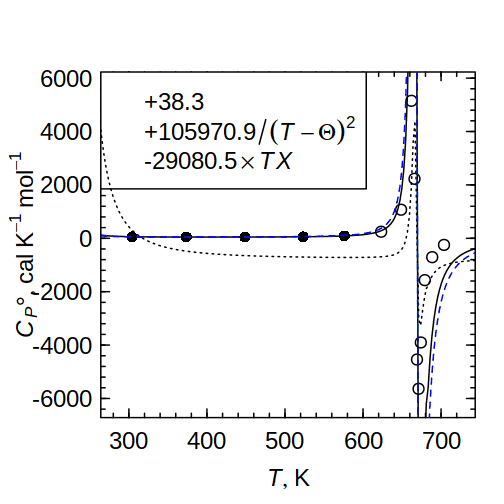Heat capacity of aqueous methane.

We can use `EOSplot()` to plot the data and fitted lines and show the coefficients in the legend. The solid line shows the fit to the lower-temperature data. The fit to all data, represented by the dotted line, doesn’t capture the low-temperature trends in the data.

``````EOSplot(Cpdat, coefficients = round(Cplm_low\$coefficients, 1))
EOSplot(Cpdat, coeficients = Cplm_high, add = TRUE, lty = 3)
PS01_data <- EOScoeffs("CH4", "Cp")
EOSplot(Cpdat, coefficients = PS01_data, add = TRUE, lty=2, col="blue")``````

Be aware that the lines shown by `EOSplot()` are calculated for a single pressure only, despite the temperature- and pressure-dependence of the data and regressions.

`EOScoeffs()` is a small function that is used to retrieve the HKF parameters in the database in CHNOSZ. The dashed blue line shows calculated values for methane using these parameters, which are from Plyasunov and Shock (2001Plyasunov AV, Shock EL. 2001. Correlation strategy for determining the parameters of the revised Helgeson-Kirkham-Flowers model for aqueous nonelectrolytes. Geochimica et Cosmochimica Acta 65(21): 3879–3900. doi: 10.1016/S0016-7037(01)00678-0). Compare the database values with the regressed values shown in the legend of figure above. Some differences are expected as the values in the database are derived from different regression techniques applied to different sets of data:

``EOScoeffs("CH4", "Cp")``
``````## (Intercept)  invTTheta2      TXBorn
##       40.87    64500.00   -40000.00``````

## Setting the value of omega

Given both high-temperature volumetric and calorimetric data for neutral species, the effective value of ω is most reliably regressed from the latter (Schulte et al., 2001Schulte MD, Shock EL, Wood RH. 2001. The temperature dependence of the standard-state thermodynamic properties of aqueous nonelectrolytes. Geochimica et Cosmochimica Acta 65(21): 3919–3930. doi: 10.1016/S0016-7037(01)00717-7). Let’s regress volumetric data using a value of omega taken from the heat capacity regression. First, read the data from Hnědkovský et al. (1996Hnědkovský L, Wood RH, Majer V. 1996. Volumes of aqueous solutions of CH4, CO2, H2S, and NH3 at temperatures from 298.15 K to 705 K and pressures to 35 MPa. Journal of Chemical Thermodynamics 28(2): 125–142. doi: 10.1006/jcht.1996.0011).

``````file <- system.file("extdata/cpetc/V.CH4.HWM96.csv", package = "CHNOSZ")
Vdat\$P <- convert(Vdat\$P, "bar")``````

Compressibilities of species (measured or estimated) are implied by the full set of HKF volumetric parameters (a1, a2, a3, a4). In this example we model volumes at nearly constant P. Therefore, we can use a simpler equation for V° written in terms of the “isobaric fit parameters” (Tanger and Helgeson 1988, p. 35) σ and ξ, together with the solvation contribution that depends on the Q Born function:

V° = σ + ξ / (T - θ) - ωQ

`EOSvar()` actually returns the negative of Q, so the omega symbol here carries no negative sign.

Now we calculate the Q Born function using `EOSvar()` and multiply by ω (from the heat capacity regression) to get the solvation volume at each experimental temperature and pressure. Subtract the solvation volume from the experimental volumes and create a new data frame holding the calculated “non-solvation” volume.

Because we are dealing with volumes, the units of ω are converted according to 1 cal = 41.84 cm3 bar.

``````QBorn <- EOSvar("QBorn", T = Vdat\$T, P = Vdat\$P)
Vomega <- convert(Cplm_low\$coefficients[["TXBorn"]], "cm3bar")
V_sol <- Vomega * QBorn
V_non <- Vdat\$V - V_sol
Vdat_non <- data.frame(T = Vdat\$T, P = Vdat\$P, V = V_non)``````

Next, regress the non-solvation volume using the non-solvation terms in the HKF model. As with CP°, also get the values of the parameters from the database for comparison with the regression results.

``````var <- "invTTheta"
Vnonlm <- EOSregress(Vdat_non, var, T.max = 450)
Vcoeffs <- round(c(Vnonlm\$coefficients, QBorn = Vomega), 1)
Vcoeffs_database <- EOScoeffs("CH4", "V")``````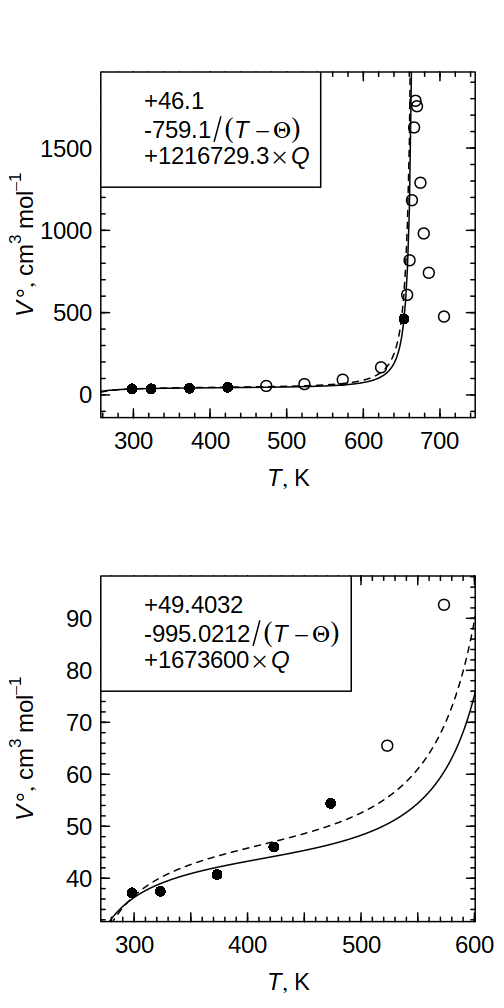Volume of aqueous methane.

Finally, plot the data and regressions. The first plot shows all the data, and the second the low-temperature subset (T < 600 K). The solid line is the two-term fit for σ and ξ (using ω from the heat capacity regression), and the dashed line shows the volumes calculated using the parameters in the database. The plot legends give the parameters from the two-term fit (first plot), or from the database (second plot).

``````par(mfrow = c(2, 1))
# plot 1
EOSplot(Vdat, coefficients = Vcoeffs)
EOSplot(Vdat, coefficients = Vcoeffs_database, add = TRUE, lty = 2)
# plot 2
EOSplot(Vdat, coefficients = Vcoeffs_database, T.plot = 600, lty = 2)
EOSplot(Vdat, coefficients = Vcoeffs, add = TRUE)``````

The equation for V° provides a reasonable approximation of the trend of lowest-temperature data (T < 450 K). However, the equation does not closely reproduce the trend of higher-temperature V° data (T < 600 K), nor behavior in the critical region. Because of these issues, some researchers are exploring alternatives to the HKF model for aqueous nonelectrolytes. (See also an example in `?EOSregress`.)

# An example for charged species

For this example, let’s generate synthetic data for Na+ using its parameters in the database. In the call to `subcrt()` below, `convert = FALSE` means to take T in units of K.

``````T <- convert(seq(0, 600, 50), "K")
P <- 1000
prop.PT <- subcrt("Na+", T = T, P = P, grid = "T", convert = FALSE)\$out[]
Nadat <- prop.PT[, c("T", "P", "Cp")]``````

As noted above, ω for electrolytes is not a constant. What happens if we apply the constant-ω model anyway, knowing it’s not applicable (especially at high temperature)?

``````var <- c("invTTheta2", "TXBorn")
Nalm <- EOSregress(Nadat, var, T.max = 600)
EOSplot(Nadat, coefficients = Nalm\$coefficients, fun.legend = NULL)``````
```## EOSplot: plotting line for P=1000 bar
```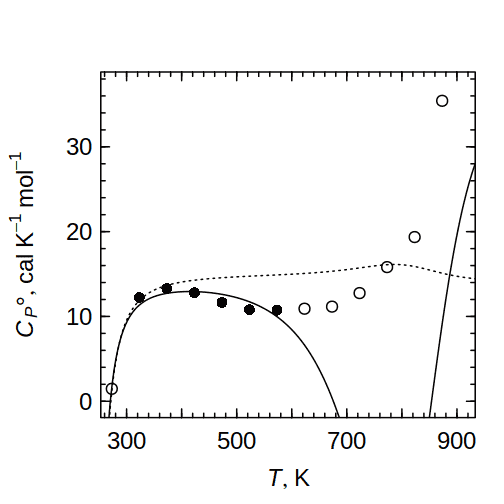Heat capacity of Na+ (inapplicable: constant ω).

``EOSplot(Nadat, add = TRUE, lty = 3)``
```## EOSplot: plotting line for P=1000 bar
```

As before, the solid line is a fit to relatively low-temperature (T < 600 K) data, and the dotted line a fit to the entire temperature range of the data. The fits using constant ω are clearly not acceptable.

There is, however, a way out. A different variable, `Cp_s_var`, can be used to specify the calculation of the “solvation” heat capacity in the HKF equations using the temperature- and pressure-dependent corrections for charged species. To use this variable, the values of ωPr,Tr (omega at the reference temperature and pressure) and Z (charge) must be given, in addition to T and P. Of course, right now we don’t know the value of ωPr,Tr—it is the purpose of the regression to find it! But we can make a first guess using the value of ω found above.

``````var1 <- c("invTTheta2", "Cp_s_var")
omega.guess <- coef(Nalm)``````

Then, we can use an iterative procedure that refines successive guesses of ωPr,Tr. The convergence criterion is measured by the difference in sequential regressed values of ω.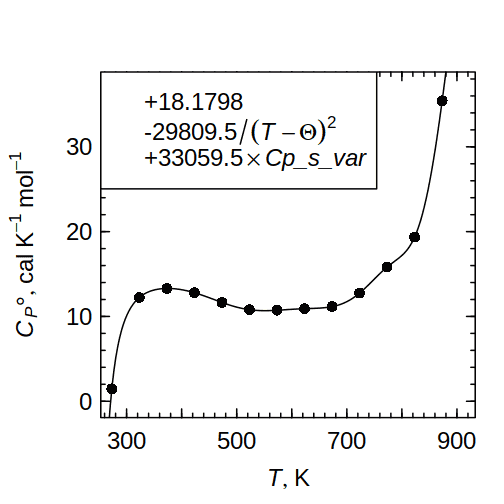Heat capacity of Na+ (variable ω).

``````diff.omega <- 999
while(abs(diff.omega) > 1) {
Nalm1 <- EOSregress(Nadat, var1, omega.PrTr = tail(omega.guess, 1), Z = 1)
omega.guess <- c(omega.guess, coef(Nalm1))
diff.omega <- tail(diff(omega.guess), 1)
}
EOSplot(Nadat, coefficients = signif(coef(Nalm1), 6),
omega.PrTr = tail(omega.guess, 1), Z = 1)``````
```## EOSplot: plotting line for P=1000 bar
```
``EOScoeffs("Na+", "Cp")``
``````## (Intercept)  invTTheta2      TXBorn
##       18.18   -29810.00    33060.00``````

Alrighty! We managed to obtain HKF coefficients from synthetic data for Na+. The regressed values of the HKF coefficients (shown in the plot legend) are very close to the database values (printed by the call to `EOScoeffs()`) used to generate the synthetic data.

## Doing it for volume

Just like above, but using synthetic V° data. Note that the regressed value of ω has volumetric units (cm3 bar/mol), while `omega.PrTr` is in caloric units (cal/mol). Compared to CP°, the regression of V° is very finicky. Given a starting guess of ωPr,Tr of 1400000 cm3 bar/mol, the iteration converges on 1394890 instead of the “true” database value of 1383230 (represented by dashed line in the plot).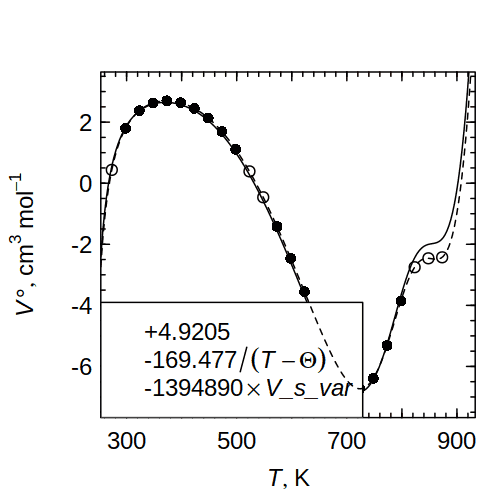Volume of Na+ (variable ω).

``````T <- convert(seq(0, 600, 25), "K")
P <- 1000
prop.PT <- subcrt("Na+", T = T, P = P, grid = "T", convert = FALSE)\$out[]
NaVdat <- prop.PT[, c("T", "P", "V")]
var1 <- c("invTTheta", "V_s_var")
omega.guess <- 1400000
diff.omega <- 999
while(abs(diff.omega) > 1) {
NaVlm1 <- EOSregress(NaVdat, var1,
omega.PrTr = tail(convert(omega.guess, "calories"), 1), Z = 1)
omega.guess <- c(omega.guess, coef(NaVlm1))
diff.omega <- tail(diff(omega.guess), 1)
}
EOSplot(NaVdat, coefficients = signif(coef(NaVlm1), 6),
omega.PrTr = tail(convert(omega.guess, "calories"), 1), Z = 1,
fun.legend = "bottomleft")
coefficients <- EOScoeffs("Na+", "V", P = 1000)
names(coefficients) <- "V_s_var"
EOSplot(NaVdat, coefficients = coefficients, Z = 1, add = TRUE, lty = 2,
omega.PrTr = convert(coefficients["V_s_var"], "calories"))``````

# Making a pseudospecies: H4SiO4

Some mineral stability diagrams use the activity of H4SiO4 as a variable. However, the primary species for dissolved silica in CHNOSZ is SiO2(aq). As recommended by Wolery and Jové Colón (2017Wolery TJ, Jové Colón CF. 2017. Chemical thermodynamic data. 1. The concept of links to the chemical elements and the historical development of key thermodynamic data. Geochimica et Cosmochimica Acta 213(Supplement C): 635–676. doi: 10.1016/j.gca.2016.09.028), let us use data for SiO2(aq) from Apps and Spycher (2004Apps J, Spycher N. 2004. Data qualification for thermodynamic data used to support THC calculations. Las Vegas, NV: Bechtel SAIC Company, LLC. Report No.: ANL-NBS-HS-000043 REV 00 (DOC.20041118.0004).), which gives a higher solubility of quartz compared to values from Shock et al. (1989Shock EL, Helgeson HC, Sverjensky DA. 1989. Calculation of the thermodynamic and transport properties of aqueous species at high pressures and temperatures: Standard partial molal properties of inorganic neutral species. Geochimica et Cosmochimica Acta 53(9): 2157–2183. doi: 10.1016/0016-7037(89)90341-4) that are loaded by default in the package:

``add.obigt("AS04")``

The pseudo-reaction with zero properties, H4SiO4 = SiO2 + 2 H2O, defines the properties of the pseudospecies H4SiO4. First we go about calculating the properties of SiO2 + 2 H2O. We do this over a range of T and P, but include many points near 25 °C to improve the fit of the regression in that region:

``````s_25C <- subcrt(c("SiO2", "H2O"), c(1, 2), T = 25)\$out
s_near25 <- subcrt(c("SiO2", "H2O"), c(1, 2), T = seq(20, 30, length.out=50))\$out
s_lowT <- subcrt(c("SiO2", "H2O"), c(1, 2), T = seq(0, 100, 10))\$out
s_Psat <- subcrt(c("SiO2", "H2O"), c(1, 2))\$out
s_P500 <- subcrt(c("SiO2", "H2O"), c(1, 2), T = seq(0, 1000, 100), P = 500)\$out
s_P1000 <- subcrt(c("SiO2", "H2O"), c(1, 2), T = seq(0, 1000, 100), P = 1000)\$out``````

Now we can start making the new species, with thermodynamic properties calculated at 25 °C:

``````mod.obigt("calc-H4SiO4", formula = "H4SiO4", ref1 = "this_vignette",
date = today(), G = s_25C\$G, H = s_25C\$H, S = s_25C\$S,
Cp = s_25C\$Cp, V = s_25C\$V, z = 0)``````
```## mod.obigt: added calc-H4SiO4(aq) with energy units of cal
```
``##  3374``

To prepare for the regression, combine the calculated data and convert °C to K:

``````substuff <- rbind(s_near25, s_lowT, s_Psat, s_P500, s_P1000)
substuff\$T <- convert(substuff\$T, "K")``````

Now let’s run a CP° regression and update the new species with the regressed HKF coefficients. Note that we apply order-of-magnitude scaling to the coefficients (see `?thermo`):

``````Cpdat <- substuff[, c("T", "P", "Cp")]
var <- c("invTTheta2", "TXBorn")
Cplm <- EOSregress(Cpdat, var)
Cpcoeffs <- Cplm\$coefficients
mod.obigt("calc-H4SiO4", c1 = Cpcoeffs,
c2 = Cpcoeffs/10000, omega = Cpcoeffs/100000)``````
```## mod.obigt: updated calc-H4SiO4(aq)
```

Let’s get ready to regress V° data. We use the strategy shown above to calculate non-solvation volume using ω from the CP° regression:

``````Vdat <- substuff[, c("T", "P", "V")]
QBorn <- EOSvar("QBorn", T = Vdat\$T, P = Vdat\$P)
Vomega <- convert(Cplm\$coefficients[["TXBorn"]], "cm3bar")
V_sol <- Vomega * QBorn
V_non <- Vdat\$V - V_sol
Vdat\$V <- V_non``````

Here’s the V° regression for the pseudospecies. We specify the variables for the a1, a2, a3, and a4 terms in the HKF equations.

``````var <- c("invPPsi", "invTTheta", "invPPsiTTheta")
Vlm <- EOSregress(Vdat, var)
Vcoeffs <- convert(Vlm\$coefficients, "calories")
mod.obigt("calc-H4SiO4", a1 = Vcoeffs*10, a2 = Vcoeffs/100,
a3 = Vcoeffs, a4 = Vcoeffs/10000)``````
```## mod.obigt: updated calc-H4SiO4(aq)
```

We just calculated the properties of the H4SiO4 pseudospecies. For comparison, the database in CHNOSZ contains `H4SiO4` with parameters from Stefánsson (2001Stefánsson A. 2001. Dissolution of primary minerals of basalt in natural waters. I. Calculation of mineral solubilities from 0°C to 350°C. Chemical Geology 172: 225–250. doi: 10.1016/S0009-2541(00)00263-1):

``info(info(c("calc-H4SiO4", "H4SiO4")))``
``````##             name abbrv formula state          ref1 ref2      date E_units       G       H       S      Cp      V      a1       a2       a3       a4      c1      c2    omega Z
## 3374 calc-H4SiO4  <NA>  H4SiO4    aq this_vignette <NA>  2.Aug.19     cal -312915 -348813 44.5529 13.7338 51.607 2.56914 -3289.32 -63.5736 156985.0 71.3306 -266424 33482.60 0
## 3373      H4SiO4  <NA>  H4SiO4    aq         Ste01 <NA> 31.Aug.06     cal -312920 -348676 45.1004 15.0096 52.300 1.87299 -2126.00  18.6200 -12000.5 58.0306 -207899  8690.25 0``````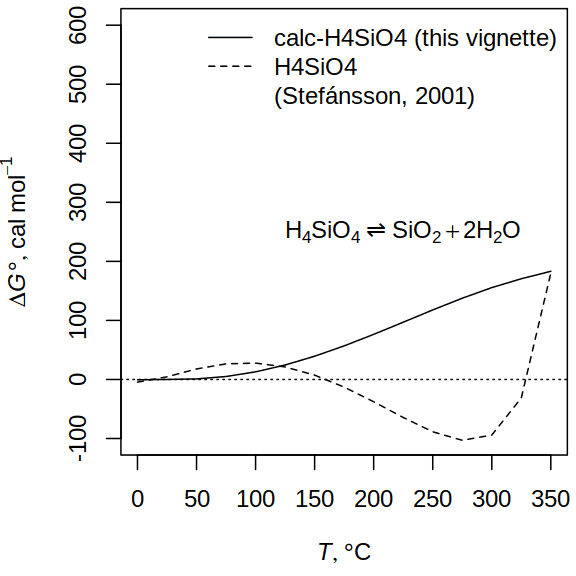Comparison of H4SiO4 pseudospecies.

Let’s compare `H4SiO4` from Stefánsson (2001) and the `calc-H4SiO4` we just made with the calculated properties of SiO2 + 2 H2O as a function of temperature:

``````s1 <- subcrt(c("calc-H4SiO4", "SiO2", "H2O"), c(-1, 1, 2))
plot(s1\$out\$T, s1\$out\$G, type = "l", ylim = c(-100, 600),
xlab = axis.label("T"), ylab = axis.label("DG0"))
s2 <- subcrt(c("H4SiO4", "SiO2", "H2O"), c(-1, 1, 2))
lines(s2\$out\$T, s2\$out\$G, lty = 2)
abline(h = 0, lty = 3)
legend("topright", legend = c("calc-H4SiO4 (this vignette)",
"H4SiO4", "(Stef\u00e1nsson, 2001)"), lty = c(1, 2, NA), bty = "n")
text(225, 250, describe.reaction(s1\$reaction))``````

Ideally, the lines would be horizontal with a y-interecept of 0. However, both the `calc-H4SiO4` we made here and the `H4SiO4` from Stefánsson (2001) show deviations that increase at higher temperatures. While they are not quite negligible, these deviations are comparatively small. For example, the almost 200 cal/mol offset in ΔG° at 350 °C corresponds to a difference in logK of only -0.07.

The following example uses the `H4SiO4` from Stefánsson (2001) to make an activity diagram for the K2O-Al2O3-SiO2-H2O system. This is similar to diagrams found, for example, on p. 361 of Garrels and Christ, 1965 and Figure 3 of Steinmann et al., 1994, but is quantitatively a closer match to the diagram in the User’s Guide to PHREEQC.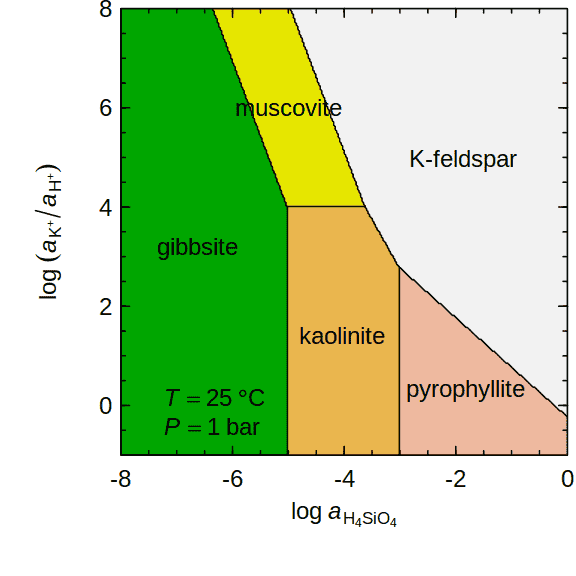Activity diagram for K2O-Al2O3-SiO2-H2O.

``````basis(c("Al+3", "H4SiO4", "K+", "H2O", "H+", "O2"))
species(c("gibbsite", "muscovite", "kaolinite", "pyrophyllite", "K-feldspar"))
a <- affinity(H4SiO4 = c(-8, 0, 300), `K+` = c(-1, 8, 300))
diagram(a, ylab = ratlab("K+"), fill = "terrain", yline = 1.7)
legend("bottomleft", describe.property(c("T", "P"), c(25, 1)), bty = "n")``````